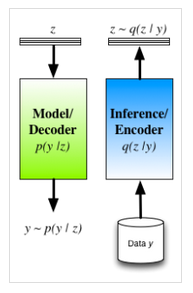# 广义的降噪自动编码器（GDAEs）

$$\textrm{Perturbation:}\quad \mathbf{y}’ \sim\mathcal{C}(\mathbf{y}’|\mathbf{y})$$

$$\textrm{Encoder:}\quad \mathbf{z(y’)} = f_\phi (\mathbf{y’})\qquad\textrm{Decoder:}\quad \mathbf{y} \approx g_\theta (\mathbf{z})$$

$$\textrm{Objective:}\quad\mathcal{L}_{DAE} = \log p(\mathbf{y} |\mathbf{z})$$

$$\quad\mathcal{L}_{GDAE} = \log p(\mathbf{y} |\mathbf{z}) – \lambda \mathcal{R}(\mathbf{y, y’})$$

GDAEs方法的原理是观测空间上的扰动能增强编码器结果$\mathbf{z}$的稳健性和不敏感性。使用GDAEs时，我们需要注意两个关键的问题：1）如何选择一个符合实际的噪化过程；2）如何选择合适的调整函数$\mathcal{R}(\cdot)$

# 分离模型与推断$$\mathcal{L}_{VFE} = \mathbb{E}_{q(\mathbf{z})}[ \log p(\mathbf{y} | \mathbf{z})] – KL[q(\mathbf{z}) \|p(\mathbf{z})]$$

1）不同于考虑观测值上的扰动，该公式考虑在隐藏值上通过$\mathbf{z}$的先验分布$p\mathbf{(z)}$获得的扰动。这时隐藏层变量是随机隐变量，而自编码器是一个可以用来直接抽样的生成模型。

2）编码器$q\mathbf{(z|y)}$用来近似潜变量的真实后验分布$p\mathbf{(z|y)}$

3）我们现在可以从理论上解释GDAE目标函数中引入的惩罚函数。与其人为设计惩罚项，我们更应该推导出这个惩罚函数的形式应该是先验概率与编码器分布之间的KL距离。

# 总结

1. Pascal Vincent, Hugo Larochelle, Yoshua Bengio, Pierre-Antoine Manzagol,Extracting and composing robust features with denoising autoencoders, Proceedings of the 25th international conference on Machine learning, 2008
2. Yoshua Bengio, Li Yao, Guillaume Alain, Pascal Vincent, Generalized denoising auto-encoders as generative models, Advances in Neural Information Processing Systems, 2013
3. Pascal Vincent, Hugo Larochelle, Yoshua Bengio, Pierre-Antoine Manzagol,Extracting and composing robust features with denoising autoencoders, Proceedings of the 25th international conference on Machine learning, 2008
4. Danilo Jimenez Rezende, Shakir Mohamed, Daan Wierstra, Stochastic Backpropagation and Approximate Inference in Deep Generative Models, Proceedings of The 31st International Conference on Machine Learning, 2014
5. Diederik P Kingma, Max Welling, Auto-encoding variational bayes, arXiv preprint arXiv:1312.6114, 2014
6. Danilo Jimenez Rezende, Shakir Mohamed, Daan Wierstra, Stochastic Backpropagation and Approximate Inference in Deep Generative Models, Proceedings of The 31st International Conference on Machine Learning, 2014
7. Diederik P Kingma, Shakir Mohamed, Danilo Jimenez Rezende, Max Welling, Semi-supervised learning with deep generative models, Advances in Neural Information Processing Systems, 2014
8. Karol Gregor, Ivo Danihelka, Alex Graves, Daan Wierstra, DRAW: A Recurrent Neural Network For Image Generation, arXiv preprint arXiv:1502.04623, 2015